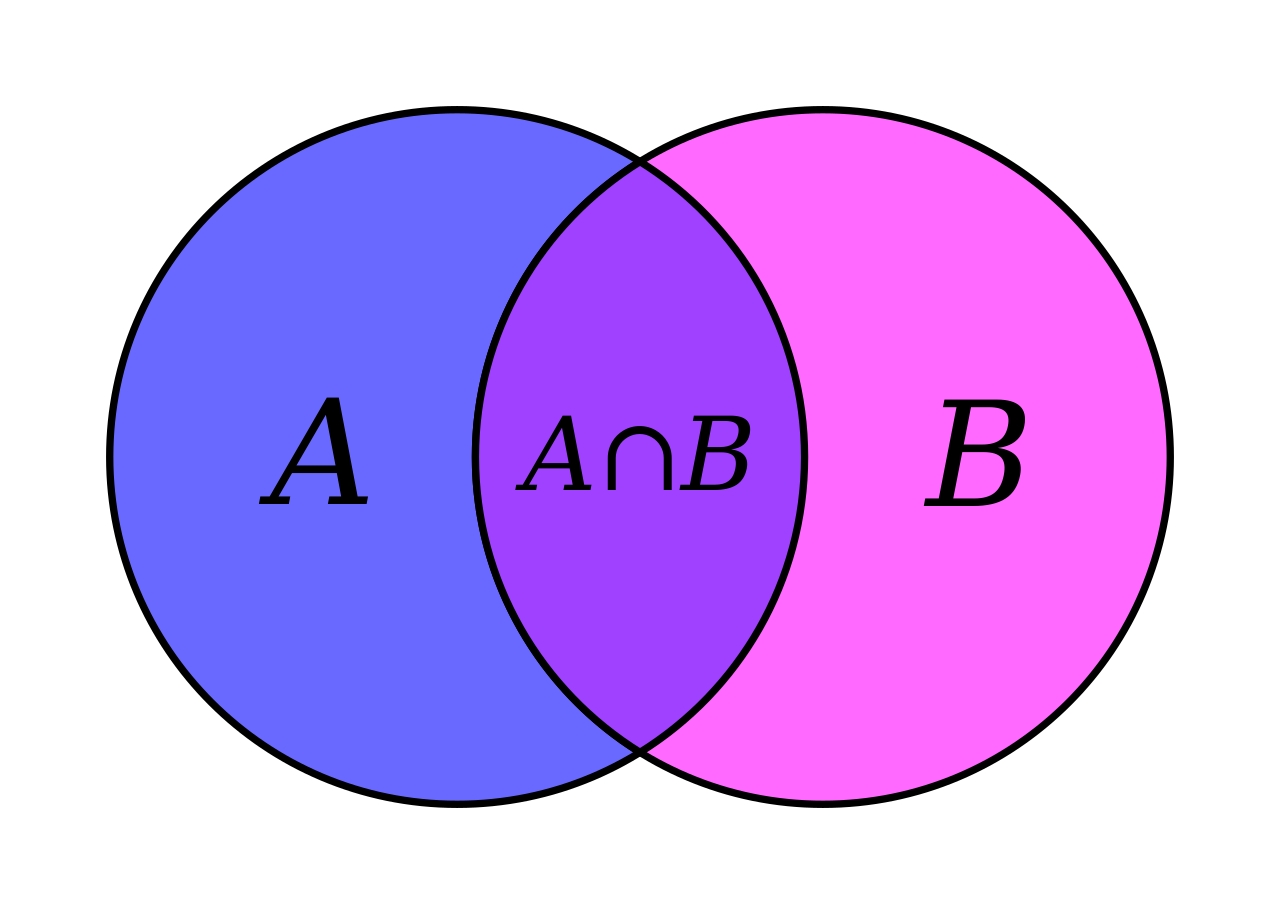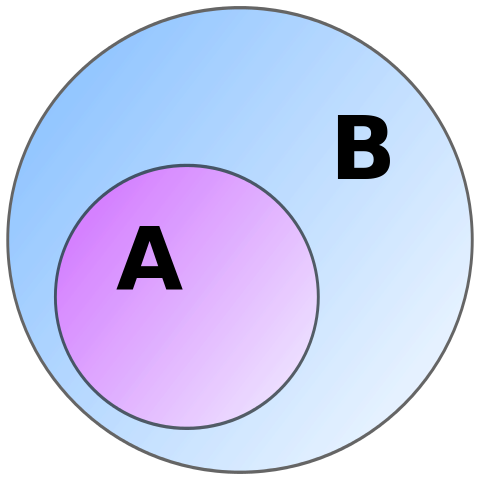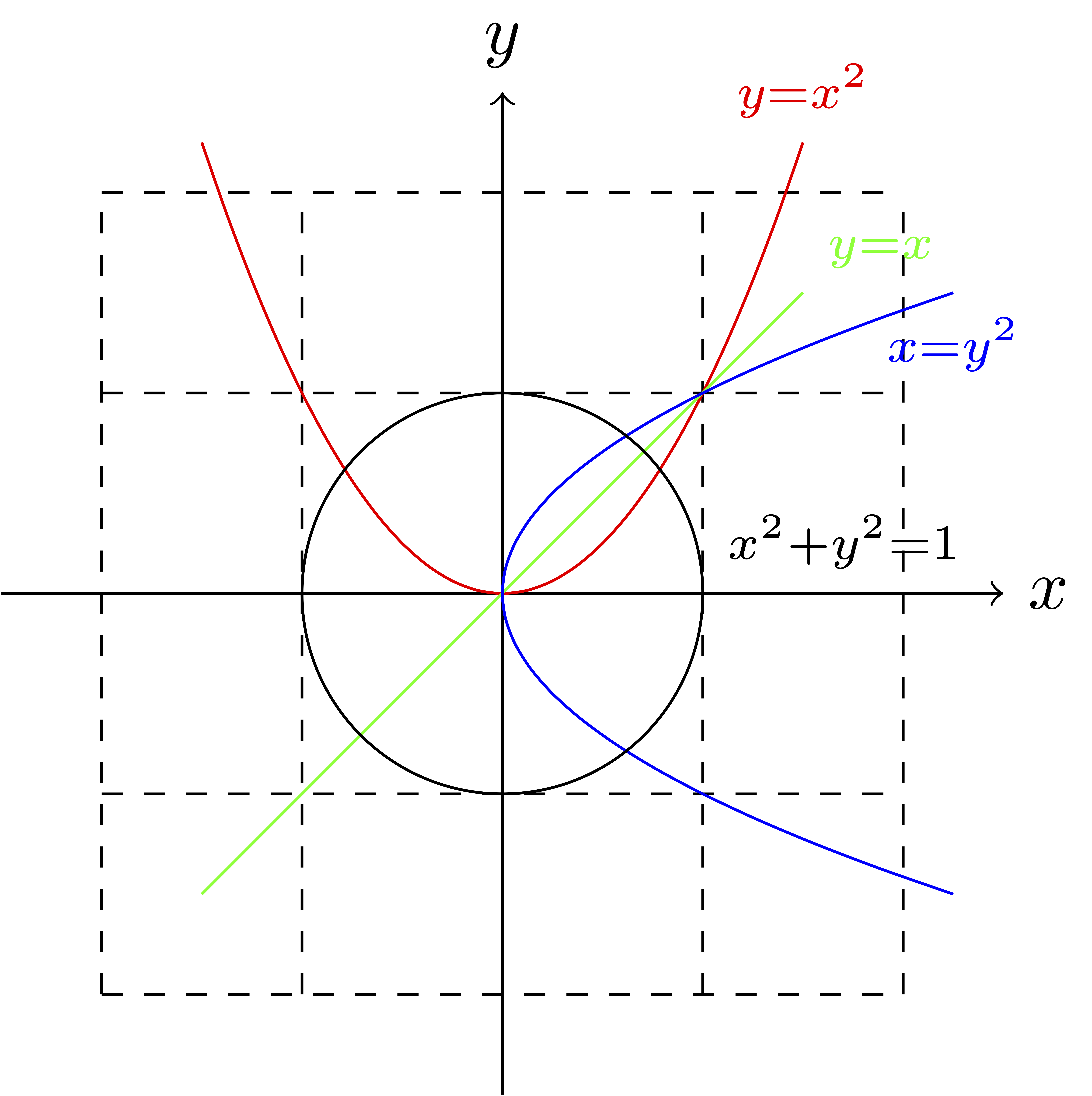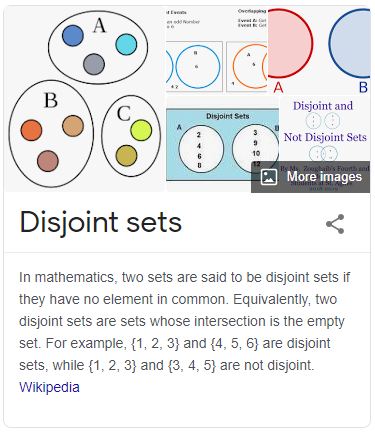Closed Loop Interval OntologyCLOSED LOOP INTERVAL ONTOLOGY        The Digital Integration of Conceptual FormTzimTzum/Kaballah | Loop definition | Home | ORIGINPlease sign in or register Email * Password * Searches selected display Universal conceptual form Aligning the vision Evolving and coalescing Why we do this Ethics / governance / science Homeostatic governance Idealized democracy Reconciliation and integration Holistic view on alternatives Definitions and alternatives Compare alternatives How do we know? How meaning is created Foundational recursive definition Spectrum of levels The closed loop ensemble contains all primary definitions Dimensions of set theory What is a number? Topology of sets How are they constructed? Primary terms Closed Loop framework Hierarchical models Euclid in digital space Foundational method Compositional semantics How it works The integrated science of mind What does it mean? Core terms Constructive elements and building blocks Preserving data under transformation In the beginning Domain and universal A design proposal Mapping the grid From other sources Foundational computation Perfecting political balance Simultaneity or sequence Concrete symbolic representation Science, philosophy and math are defined in concepts Emerging from a pointSet
Dimensions of set theory

 The foundational concept of mathematics is "set". In mathematics, a set is a collection of distinct elements. The elements that make up a set can be any kind of things: people, letters of the alphabet, numbers, points in space, lines, other geometrical shapes, variables, or even other sets. Two sets are equal if and only if they have precisely the same elements. Sets are ubiquitous in modern mathematics. The subject called set theory is part of the foundations of mathematics.Set theory (all) A set is a collection of objects Sat, Mar 20, 2021 Reference Set theory is a branch of mathematical logic that studies sets, which informally are collections of objects. Although any type of object can be collected into a set, set theory is applied most often to objects that are relevant to mathematics. The language of set theory can be used to define nearly all mathematical objects. The modern study of set theory was initiated by Georg Cantor and Richard Dedekind in the 1870s. After the discovery of paradoxes in naïve set theory, such as Russell's paradox, numerous axiom systems were proposed in the early twentieth century, of which the Zermelo–Fraenkel axioms, with or without the axiom of choice, are the best-known. Set theory is commonly employed as a foundational system for mathematics, particularly in the form of Zermelo–Fraenkel set theory with the axiom of choice. Beyond its foundational role, set theory is a branch of mathematics in its own right, with an active research community. Contemporary research into set theory includes a diverse collection of topics, ranging from the structure of the real number line to the study of the consistency of large cardinals.

 Set theory and geometry We want to explore deriving the concept of set and "members of a set" from geometry -- from what used to be Euclid, to what we are now exploring as "digital geometry" Euclid defines a "line" -- we want to strengthen that definition Lines define the boundaries of set and what is contained within set -- the boundaries between the objects -- the members of the set -- when they are ordered ** And of course -- there are Venn diagrams -- circular boundaries around things they contain Fri, Apr 2, 2021

 Set theory (naïve) Naive set theory is any of several theories of sets used in the discussion of the foundations of mathematics. Unlike axiomatic set theories, which are defined using formal logic, naive set theory is defined informally, in natural language. It describes the aspects of mathematical sets familiar in discrete mathematics (for example Venn diagrams and symbolic reasoning about their Boolean algebra), and suffices for the everyday use of set theory concepts in contemporary mathematics. Sets are of great importance in mathematics; in modern formal treatments, most mathematical objects (numbers, relations, functions, etc.) are defined in terms of sets. Naive set theory suffices for many purposes, while also serving as a stepping-stone towards more formal treatments. ** Some important sets There are some ubiquitous sets for which the notation is almost universal. Some of these are listed below. In the list, a, b, and c refer to natural numbers, and r and s are real numbers. Natural numbers are used for counting. A blackboard bold capital N often represents this set. Integers appear as solutions for x in equations like x + a = b. A blackboard bold capital Z often represents this set (from the German Zahlen, meaning numbers). Rational numbers appear as solutions to equations like a + bx = c. A blackboard bold capital Q often represents this set (for quotient, because R is used for the set of real numbers). Algebraic numbers appear as solutions to polynomial equations (with integer coefficients) and may involve radicals and certain other irrational numbers. A Q with an overline often represents this set. The overline denotes the operation of algebraic closure. Real numbers represent the "real line" and include all numbers that can be approximated by rationals. These numbers may be rational or algebraic but may also be transcendental numbers, which cannot appear as solutions to polynomial equations with rational coefficients. A blackboard bold capital R often represents this set. Fri, Mar 19, 2021 Reference Naive set theory is any of several theories of sets used in the discussion of the foundations of mathematics. Unlike axiomatic set theories, which are defined using formal logic, naive set theory is defined informally, in natural language. It describes the aspects of mathematical sets familiar in discrete mathematics (for example Venn diagrams and symbolic reasoning about their Boolean algebra), and suffices for the everyday use of set theory concepts in contemporary mathematics. Sets are of great importance in mathematics; in modern formal treatments, most mathematical objects (numbers, relations, functions, etc.) are defined in terms of sets. Naive set theory suffices for many purposes, while also serving as a stepping-stone towards more formal treatments.

 Set theory (axiomatic) Elementary set theory can be studied informally and intuitively, and so can be taught in primary schools using Venn diagrams. The intuitive approach tacitly assumes that a set may be formed from the class of all objects satisfying any particular defining condition. This assumption gives rise to paradoxes, the simplest and best known of which are Russell's paradox and the Burali-Forti paradox. Axiomatic set theory was originally devised to rid set theory of such paradoxes. Fri, Mar 19, 2021

 Set theory (class) In set theory and its applications throughout mathematics, a class is a collection of sets (or sometimes other mathematical objects) that can be unambiguously defined by a property that all its members share. The precise definition of "class" depends on foundational context. In work on Zermelo–Fraenkel set theory, the notion of class is informal, whereas other set theories, such as von Neumann–Bernays–Gödel set theory, axiomatize the notion of "proper class", e.g., as entities that are not members of another entity. A class that is not a set (informally in Zermelo–Fraenkel) is called a proper class, and a class that is a set is sometimes called a small class. For instance, the class of all ordinal numbers, and the class of all sets, are proper classes in many formal systems. In Quine's set-theoretical writing, the phrase "ultimate class" is often used instead of the phrase "proper class" emphasising that in the systems he considers, certain classes cannot be members, and are thus the final term in any membership chain to which they belong. Outside set theory, the word "class" is sometimes used synonymously with "set". This usage dates from a historical period where classes and sets were not distinguished as they are in modern set-theoretic terminology. Many discussions of "classes" in the 19th century and earlier are really referring to sets, or perhaps rather take place without considering that certain classes can fail to be sets. Fri, Mar 19, 2021

 Ordered set In mathematics, a total order, simple order, linear order, connex order, or full order is a binary relation on some set {\displaystyle X}X, which is antisymmetric, transitive, and a connex relation. A set paired with a total order is called a chain, a totally ordered set, a simply ordered set, a linearly ordered set, or a loset. Formally, a binary relation {\displaystyle \leq }\leq is a total order on a set {\displaystyle X}X if the following statements hold for all {\displaystyle a,b}a,b and {\displaystyle c}c in {\displaystyle X}X: Antisymmetry: If {\displaystyle a\leq b}a\leq b and {\displaystyle b\leq a}{\displaystyle b\leq a} then {\displaystyle a=b}a=b; Transitivity: If {\displaystyle a\leq b}a\leq b and {\displaystyle b\leq c}{\displaystyle b\leq c} then {\displaystyle a\leq c}{\displaystyle a\leq c}; Connexity: {\displaystyle a\leq b}a\leq b or {\displaystyle b\leq a}{\displaystyle b\leq a}. Antisymmetry eliminates uncertain cases when both {\displaystyle a}a precedes {\displaystyle b}b and {\displaystyle b}b precedes {\displaystyle a}a.:325 A relation having the connex property means that any pair of elements in the set of the relation are comparable under the relation. This also means that the set can be diagrammed as a line of elements, giving it the name linear.:330 The connex property also implies reflexivity, i.e., a ? a. Therefore, a total order is also a (special case of a) partial order, as, for a partial order, the connex property is replaced by the weaker reflexivity property. An extension of a given partial order to a total order is called a linear extension of that partial order. Sat, Mar 20, 2021

 Partially ordered set What we seem to be seeing in the notion of a "partially ordered set" is that the "pure linear order" proceeds in descending/vertical levels, but there may be branching between the levels, which extends in a "partially horizontal" level. Another interesting topic is the subject of "ordered by inclusion" Which would seem to mean that A contains B and B contains C, therefore there is a linear ordering of these three elements, A, B, C. Thu, Mar 25, 2021 Reference In mathematics, especially order theory, a partially ordered set (also poset) formalizes and generalizes the intuitive concept of an ordering, sequencing, or arrangement of the elements of a set. A poset consists of a set together with a binary relation indicating that, for certain pairs of elements in the set, one of the elements precedes the other in the ordering. The relation itself is called a "partial order." The word partial in the names "partial order" and "partially ordered set" is used as an indication that not every pair of elements needs to be comparable. That is, there may be pairs of elements for which neither element precedes the other in the poset. Partial orders thus generalize total orders, in which every pair is comparable. Formally, a partial order is any binary relation that is reflexive (each element is comparable to itself), antisymmetric (no two different elements precede each other), and transitive (the start of a chain of precedence relations must precede the end of the chain). One familiar example of a partially ordered set is a collection of people ordered by genealogical descendancy. Some pairs of people bear the descendant-ancestor relationship, but other pairs of people are incomparable, with neither being a descendant of the other. A poset can be visualized through its Hasse diagram, which depicts the ordering relation.

 Set theory and dimensionality This is where we want to go -- to show that all elements of classical set theory can be defined in terms of dimensionality A set itself is a dimension -- because all objects in the set are not exactly identical -- or if they are, they can be ordered by the integers -- if they are not, they can be ordered by their differences The objects in (of) the set can be defined in terms of dimensionality Apples Oranges What is the differences between a set and a class? Thu, Mar 25, 2021

 Linnaeus on similarities and differences All the real knowledge which we possess depends on methods by which we distinguish the similar from the dissimilar. The greater the number of natural distinctions this method comprehends the clearer becomes our idea of things. The more numerous the objects which employ our attention the more difficult it becomes to form such a method, and the more necessary. -- Carolus Linnaeus, Genera Plantarum, 1737 We are exploring a new algebraic theory of knowledge representation based on the isomorphism of "dimension" and "ordered class". A "synthetic dimension" is defined recursively, as an ordered class of variables, themselves described in synthetic dimensions. All abstract classes can be assembled from lowest-level empirical measurements through this method All empirical measurement is defined by values in dimensions. All abstract classes are defined by composite assemblies of dimensions, and an ordered class can be shown to be algebraically isomorphic to a dimension. Thus, any high-level composite abstraction can be determinately defined bottom-up from lowest-level empirical/dimensional measurements. This approach creates a linearly recursive cascade across ascending levels of abstraction, through which any concept, category, classification, or data structure can be exactly defined, to any desired degree of accuracy/error tolerance (number of decimal places in measurement). Synthetic Dimensionality is, at the same time, a model of the foundations of mathematics (real number line), an intuitive theory of natural language semantics, an algebraic theory of classification, and a general theory of all conceptual structure. Any idea, concept, mental model, or "information structure" can be constructed in perfect detail with synthetic dimensions. Fri, Mar 26, 2021

 Set and boundary We want to generalize the notion of set in terms of dimensionality and boundary values Thu, Mar 25, 2021

Set, venn diagram, dimensionality, boundary

Set, venn diagram, dimensionality, boundary

Define the concept of set in terms of boundary and its objects in terms of dimensionality

(abstract object, concrete object)

At a minimum, a set has a boundary -- things IN the set, and things NOT IN the setCan that be specified by dimensionality?

Yes, as long as the objects are defined in terms of dimensionality

Abstract objects are defined in terms of dimensionality, and then a mapping/correlation is established by the abstract object (the word, the symbol) and the concrete object ("the actual thing" it is pointing to))

Sat, Apr 3, 2021

 Set Venn diagrams

 Abstract object, concrete object Most of the mathematics we are exploring has to do with abstract objects -- by which we mean some symbolic representation -- usually in an alphabet -- and actually existing in some medium -- such as words written on printed on a piece of paper. Is a generalization or "universal" an abstract object? I would say yes, in that its only concrete actuality/reality is that it points to a class of specific objects -- which in some cases might also be abstract or universal Fri, Mar 26, 2021 Reference In metaphysics, the distinction between abstract and concrete refers to a divide between two types of entities. Many philosophers hold that this difference has fundamental metaphysical significance. Examples of concrete objects include plants, human beings and planets while things like numbers, sets and propositions are abstract objects. There is no general consensus as to what the characteristic marks of concreteness and abstractness are. Popular suggestions include defining the distinction in terms of the difference between (1) existence inside or outside space-time, (2) having causes and effects or not, (3) having contingent or necessary existence, (4) being particular or universal and (5) belonging to either the physical or the mental realm or to neither. Despite this diversity of views, there is broad agreement concerning most objects as to whether they are abstract or concrete. So under most interpretations, all these views would agree that, for example, plants are concrete objects while numbers are abstract objects. Abstract objects are most commonly used in philosophy and semantics. They are sometimes called abstracta in contrast to concreta. The term abstract object is said to have been coined by Willard Van Orman Quine. Abstract object theory is a discipline that studies the nature and role of abstract objects. It holds that properties can be related to objects in two ways: through exemplification and through encoding. Concrete objects exemplify their properties while abstract objects merely encode them. This approach is also known as the dual copula strategy. The type–token distinction identifies physical objects that are tokens of a particular type of thing. The "type" of which it is a part is in itself an abstract object. The abstract–concrete distinction is often introduced and initially understood in terms of paradigmatic examples of objects of each kind:

 Binary relationship (math) This model show four "relations" between X and YTue, Mar 30, 2021 Reference In mathematics (specifically set theory), a binary relation over sets X and Y is a subset of the Cartesian product X × Y; that is, it is a set of ordered pairs (x, y) consisting of elements x in X and y in Y. It encodes the common concept of relation: an element x is related to an element y, if and only if the pair (x, y) belongs to the set of ordered pairs that defines the binary relation. A binary relation is the most studied special case n = 2 of an n-ary relation over sets X1, ..., Xn, which is a subset of the Cartesian product X1 × ... × Xn. An example of a binary relation is the "divides" relation over the set of prime numbers {\displaystyle \mathbb {P} }\mathbb {P} and the set of integers {\displaystyle \mathbb {Z} }\mathbb {Z} , in which each prime p is related to each integer z that is a multiple of p, but not to an integer that is not a multiple of p. In this relation, for instance, the prime number 2 is related to numbers such as ?4, 0, 6, 10, but not to 1 or 9, just as the prime number 3 is related to 0, 6, and 9, but not to 4 or 13. Binary relations are used in many branches of mathematics to model a wide variety of concepts. These include, among others: the "is greater than", "is equal to", and "divides" relations in arithmetic; the "is congruent to" relation in geometry; the "is adjacent to" relation in graph theory; the "is orthogonal to" relation in linear algebra. A function may be defined as a special kind of binary relation. Binary relations are also heavily used in computer science. A binary relation over sets X and Y is an element of the power set of X × Y. Since the latter set is ordered by inclusion (?), each relation has a place in the lattice of subsets of X × Y. Since relations are sets, they can be manipulated using set operations, including union, intersection, and complementation, and satisfying the laws of an algebra of sets. Beyond that, operations like the converse of a relation and the composition of relations are available, satisfying the laws of a calculus of relations, for which there are textbooks by Ernst Schröder, Clarence Lewis, and Gunther Schmidt. A deeper analysis of relations involves decomposing them into subsets called concepts, and placing them in a complete lattice. In some systems of axiomatic set theory, relations are extended to classes, which are generalizations of sets. This extension is needed for, among other things, modeling the concepts of "is an element of" or "is a subset of" in set theory, without running into logical inconsistencies such as Russell's paradox. The terms correspondence, dyadic relation and two-place relation are synonyms for binary relation, though some authors use the term "binary relation" for any subset of a Cartesian product X × Y without reference to X and Y, and reserve the term "correspondence" for a binary relation with reference to X and Y.
 Disjoint sets Sets with no elements in commonSun, May 30, 2021 Reference In mathematics, two sets are said to be disjoint sets if they have no element in common. Equivalently, two disjoint sets are sets whose intersection is the empty set. For example, {1, 2, 3} and {4, 5, 6} are disjoint sets, while {1, 2, 3} and {3, 4, 5} are not disjoint. A collection of more than two sets is called disjoint if any two distinct sets of the collection are disjoint.